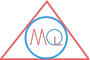# Angle and its Types

## What is an Angle?

A figure formed by joining two different rays starting from same fixed initial point is called an angle.

Example of an angle $$\angle AOB$$In the given figure, this figure is made up of two rays $$\overrightarrow{OA}$$ and $$\overrightarrow{OB}$$. The common end point of two rays is called vertex of the angle.
So, O is vertex of angle AOB.
The rays $$\overrightarrow{OA}$$ and $$\overrightarrow{OB}$$ are called the arms or sides of angle AOB.
An angle is denoted by symbol $$\angle$$.
Only capital letters of English alphabets are used to name an angle. Name of angles can be written using three or one alphabet.
Thus, we can write the above angle in figure as $$\angle AOB$$ or $$\angle BOA$$ or $$\angle O$$.
We can see from the naming that vertex is always kept at the center when written using three alphabets and only vertex when written as single alphabet.

## Measurement of angle

The unit of measuring an angle is degree.
The word degree originates from Latin word “gradius” which means “step”. It refers to a stage in an ascending or descending order.
The symbol used for degree is “$$\ ^0$$”. It is inserted on the right top of numeral.
for example, 90 degrees = $$90^0$$

## Types of angle

### 1. Acute angle

An angle which is less than $$90^o$$, is called acute angle.

Example of Acute angle### 2. Right angle

An angle which is equal to $$90^o$$, is called right angle.

Example of Right angle### 3. Obtuse angle

An angle which is greater than $$90^o$$ and less than $$180^o$$ is called obtuse angle.

Example of Obtuse angle### 4. Straight angle

An angle which is equal to $$180^o$$, is called straight angle or straight line angle.

Example of Straight angle### 5. Reflex angle

An angle which measure greater than $$180^o$$ but less than $$360^o$$ is called reflex angle.

Example of Reflex angle### 6. Complete angle

An angle is said to be complete angle if two different rays coincide with initial point after making a complete revolution.

Example of Complete angleHere, ray $$\overrightarrow{OA}$$ and ray $$\overrightarrow{OB}$$ coincide each other after making a complete revolution.
$$\angle AOB = 360^0$$

### 7. Zero angle

An angle is said to be zero angle if two different rays coincide without any revolution.

Example of Zero angleHere, ray $$\overrightarrow{OA}$$ and ray $$\overrightarrow{OB}$$ coincide $$\angle AOB$$
$$\angle AOB = 0^0$$

Note

The acute and obtuse angles are known as oblique angles.

## What are Congruent angles?

Angles having same measure are said to be congruent angles.

Example of Congruent anglesTwo angles are said to be adjacent angles if they have common vertex, a common arm and other two arms of the angles are on the opposite sides of the common arm.In the given figure, two angles $$\angle AOB$$ and $$\angle BOC$$ have a common arm OB, a common vertex O and other two arms OA and OC lie on the opposite sides of common arm OB.

### 1. Complementary angles

Two angles are said to be complementary if they form adjacent angle and sum of their measure is equal to $$90^o$$

Example of Complementary angles$$\angle AOC + \angle BOC$$
$$= 45^o + 45^o$$
$$= 90^o$$

### 2. Supplementary angles

Two angles are said to be supplementary angles if they form adjacent angles whose sum of their angles is equal to $$180^o$$

Example of Supplementary angles$$\angle ABO + \angle CBO$$
$$=120^o + 60^o$$
$$=180^o$$